# 16. Volatility Calculation (Historical)

## 16.1 – Calculating Volatility on Excel

In the previous chapter, we introduced the concept of standard deviation and how it can be used to evaluate ‘Risk or Volatility’ of a stock. Before we move any further on this topic I would like to discuss how one can calculate volatility. Volatility data is not easily available, hence its always good to know how to calculate the same yourself.

Of course in the previous chapter we looked into this calculation (recall the Billy & Mike example), we outlined the steps as follows –

1. Calculate the average
2. Calculate the deviation – Subtract the average from the actual observation
3. Square and add up all deviations – this is called variance
4. Calculate the square root of variance – this is called standard deviation

The purpose of doing this in the previous chapter was to show you the mechanics behind the standard deviation calculation. In my opinion it is important to know what really goes beyond a formula, it only enhances your insights. In this chapter however, we will figure out an easier way to calculate standard deviation or the volatility of a given stock using MS Excel. MS Excel uses the exact same steps we outlined above, just that it happens at a click of a button.

I’ll give you the border steps involved first and then elaborate on each step –

2. Calculate the daily returns
3. Use the STDEV function

So let us get to work straight away.

You can do this from any data source that you have. Some of the free and reliable data sources are NSE India website and Yahoo Finance.

I will take the data from NSE India for now. At this point I must tell you that NSE’s website is quite resourceful, and in terms of information provided, I guess NSE’s website is one of the best stock exchange websites in the world.

Anyway, in this chapter let us calculate Wipro’s volatility. To download the historical closing prices, visit – http://www.nseindia.com/products/content/equities/equities/equities.htm and click on historical data and select the search option.

Here is a snapshot where I have highlighted the search option –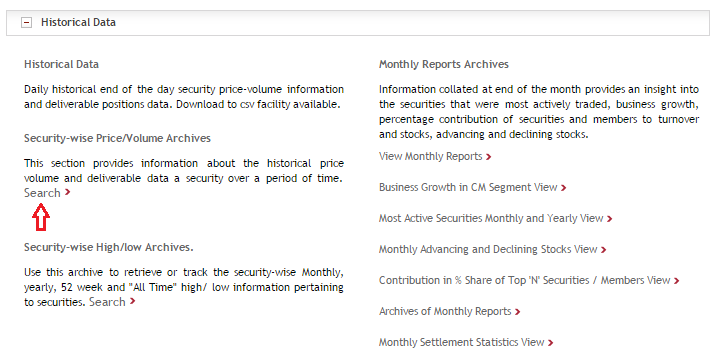Once you hit search, a set of fields open up, filling them up is quite self explanatory – just fill in the required details and hit ‘Get Data’. Do make sure you get the data for the last 1 year. The dates that I have selected here is from 22nd July 2014 to 21st July 2015.

Once you hit ‘get data’, NSE’s website will query your request and fetch you the required data. At this point you should see the following screen –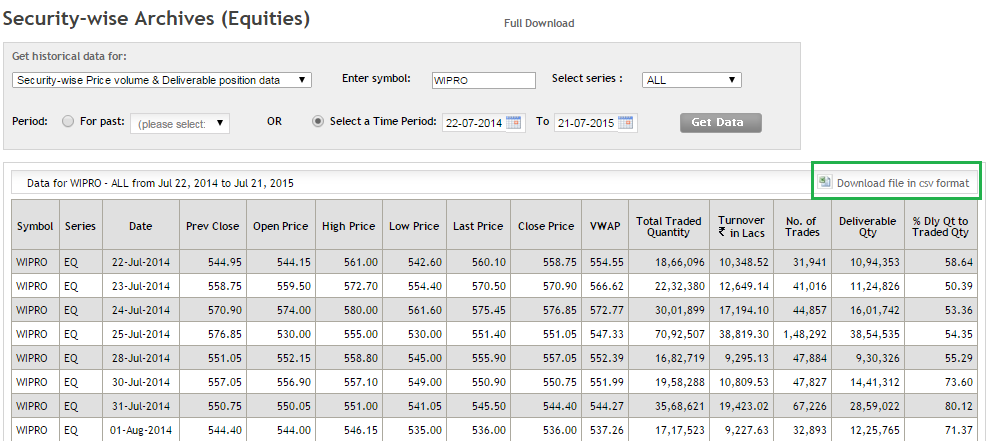Once you get this, click on ‘Download file in CSV format’ (highlighted in the green box), and that’s it.

You now have the required data on Excel. Of course along with the closing prices, you have tons of other information as well. I usually like to delete all the other unwanted data and stick to just the date and closing price. This makes the sheet look clutter free and crisp.

Here is a snapshot of how my excel sheet looks at this stage –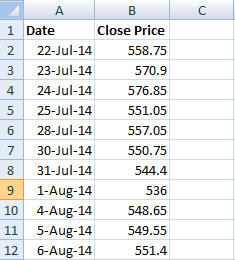Do note, I have deleted all the unnecessary information. I have retained just the date and closing prices.

Step 2 – Calculate Daily Returns

We know that the daily returns can be calculated as –

Return = (Ending Price / Beginning Price) – 1

However for all practical purposes and ease of calculation, this equation can be approximated to:

Return = LN (Ending Price / Beginning Price), where LN denotes Logarithm to Base ‘e’, note this is also called ‘Log Returns’.

Here is a snap shot showing you how I’ve calculated the daily log returns of WIPRO –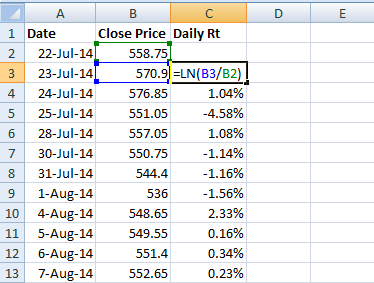I have used the Excel function ‘LN’ to calculate the long returns.

Step 3 – Use the STDEV Function

Once the daily returns are calculated, you can use an excel function called ‘STDEV’ to calculate the standard deviation of daily returns, which if you realize is the daily Volatility of WIPRO.

Note – In order to use the STDEV function all you need to do is this –

1. Take the cursor an empty cell
2. Press ‘=’
3. Follow the = sign by the function syntax i.e STDEV and open a bracket, hence the empty cell would look like =STEDEV(
4. After the open bracket, select all the daily return data points and close the bracket
5. Press enter

Here is the snapshot which shows the same –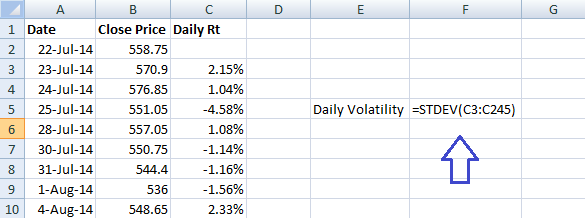Once this is done, Excel will instantly calculate the daily standard deviation aka volatility of WIPRO for you. I get the answer as 0.0147 which when converted to a percentage reads as 1.47%.

This means the daily volatility of WIPRO is 1.47% !

The value we have calculated is WIPRO’s daily volatility, but what about its annual volatility?

Now here is a very important convention you will have to remember – in order to convert the daily volatility to annual volatility just multiply the daily volatility number with the square root of time.

Likewise to convert the annual volatility to daily volatility, divide the annual volatility by square root of time.

So in this case we have calculated the daily volatility, and we now need WIPRO’s annual volatility. We will calculate the same here –

• Daily Volatility = 1.47%
• Time = 252
• Annual Volatility = 1.47% * SQRT (252)
• = 23.33%

In fact I have calculated the same on excel, have a look at the image below –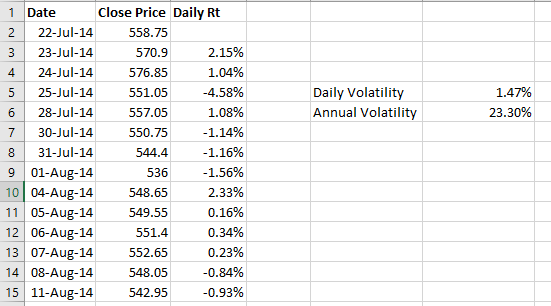So with this, we know WIPRO’s daily volatility is 1.47% and its annual volatility is about 23%.

Lets double-check these numbers with what the NSE has published on their website. NSE publishes these numbers only for F&O stocks and not other stocks. Here is the snapshot of the same –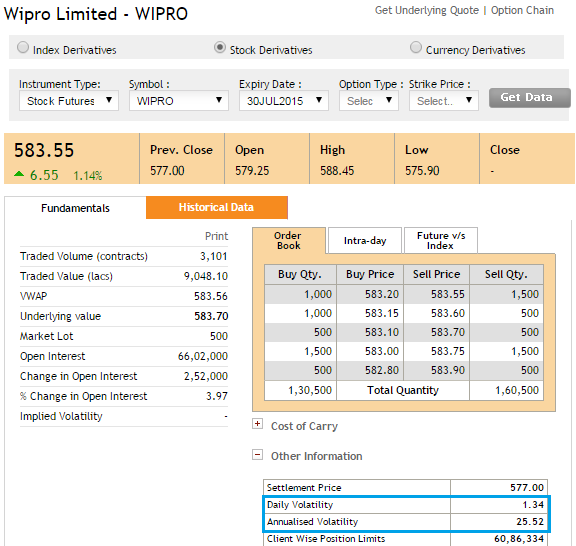Our calculation is pretty much close to what NSE has calculated – as per NSE’s calculation Wipro’s daily volatility is about 1.34% and Annualized Volatility is about 25.5%.

So why is there a slight difference between our calculation and NSE’s? – One possible reason could be that we are using spot price while NSE is using Futures price. However, I really don’t want to get into investigating why this slight difference exists. The agenda here is to know how to calculate the volatility of the security given its daily returns.

Before we wrap up this chapter, let us just do one more calculation. Assume we directly get the annual volatility of WIPRO as 25.5%, how do we figure out its daily volatility?

Like I mentioned earlier, to convert annual volatility to daily volatility you simply have to divide the annual volatility by the square root of time, hence in this particular case –

= 25.5% / SQRT (252)

= 1.60%

So far we have understood what volatility is and how to calculate the same. In the next chapter, we will understand the practical application of volatility.

Do remember we are still in the process of understanding volatility; however the final objective is to understand the options greek Vega and that really means. So please do not lose sight of our end objective.

### Key takeaways from this chapter

1. Standard Deviation represents volatility, which in turn represents risk
2. We can use NSE website to get the daily closing prices of securities
3. Daily return can be calculated as log returns
4. Log function in excel is LN
5. Daily return formula = LN (Today’s Value / Yesterday’s Value) expressed as a percentage
6. Excel function to calculate volatility is STDEV
7. Standard Deviation of daily return is equivalent of daily volatility
8. To convert daily volatility to annual volatility multiply the daily volatility by the square root of time
9. Likewise to convert annual volatility to daily volatility, divide the annual volatility by the square root of time

1.raj says:

Hi karthik, appreciate the excel calculations. makes it very simple for all of us. as far as options concerned , for e.g a put . the stock could fall to a particular price at various times and the option premium could be different at those times. i bought a put of lupin, and at one time the stock was at 1700 and the put was trading at 5. the stock went up to 1710 and when it re tested 1700 again the put was trading at 3 this time. is there a way to determine at what premium the option could trade at same stock price points at different times of the day. will you be touching upon this? thanks a ton.

•Karthik Rangappa says:

Raj, what you have quoted is a very practical situation. The main reason for this would be changes in volatility. As this mini series on Volatility evolves, I’m sure you will get the answers yourself. Please stay tuned till then 🙂

•SUHAS SHRINIVAS VAZE says:

Thanks for such a in depth practical calculation. However, can we calculate intra-day volatility ?

•Karthik Rangappa says:

You can, this requires few higher order quantitative techniques.

•Rahul Choudhary says:

Hello Karthik Sir,
Can you please tell me about those high quantitave techniques or provide me a link for the same if possible.
Thanks

Rahul

•Karthik Rangappa says:

Rahul, quants is a very broad topic, Rahul. We are discussing few techniques here – https://zerodha.com/varsity/module/trading-systems/

2.Wannbetrader says:

Fabulous :-)… Wish I was so eager to learn during my school days ,lol.

•Karthik Rangappa says:

I wish so myself, I was never a good student 🙂

3.Rajdeep says:

Hi Karthik,
I would like to congratulate you on your stellar effort in making such a complicated topic so easy to digest. If i ever succeed in trading it will only be because of you, varsity and zerodha, i say it from my heart.
I am absolutely new to trading, i am having a confusion regarding options. What i understand is the option greeks are responsible for the change in the option prices, but there is also a separate demand-supply of each option which is created by the writers and the buyers of that particular option, so what moves the option premiums the demand supply interaction like in spot market/futures or the greeks alone(the individual options demad suppy has no role to play in setting the price) or both

A 2nd question, from the current nifty option chain i see that the 8400 slightly OTM puts price have appreciated but the slightly ITM 8400 calls have fallen, does it mean the market is getting more comfortable with the idea of nifty falling below 8400 in the next 3 days, is that a case for buying slightly OTM puts, i dont have the confidence/conviction to trade, i am trying to confirm my understanding by thinking in terms of a trade. Cannot thank you enough for what you have done for me.

•Karthik Rangappa says:

Happy to read this Rajdeep…btw, if you succeed in trading (I hope you do), then its because of your heard work and nothing else. Coming to your query –

Option premium are a function of many factors, the essence of which are captured by the Greeks. For example the demand supply situation causes directional movement and thats captured by Delta. Effect of time is captured by Theta, volatility by vega etc. So Greeks capture all the necessary price variable

Regarding the 2nd question – Puts have appreciated due to two factors – Markets and fallen and volatility also has increased. You will understand and appreciate the effects of volatility over the next few chapter. Please stay tuned.

4.Rajdeep says:

Infact as i was thinking about it, i have this question for futures too, derivatives as per definition move as per the movement of the underlying, but the derivative itself is also being traded, so what determines the price of the derivative, the derivatives supply demand dynamics or the movement of the derivatives underlying or both? e.g. nifty futures is a heavily traded derivative but is the spot market single handedly determining its price ?

•Karthik Rangappa says:

A derivative derives its value from its respective underlying. Its unusual for the derivative to influence the price of the spot.

5.Pankit Shah says:

Hi Nitinji,
I have a query….right now the market is trading sideways,1 day it increases & few days it decreases….so my query is what option strategies should i employ for trading nifty options in such a volatile market…its very difficult to make money in such a market.

•Karthik Rangappa says:

Any strategy that involves a credit and benefiting from time decay would be a good I suppose. Example – short strangle, straddle etc.